# 240 373 Image Processing Montri Karnjanadecha montricoe psu

• Slides: 14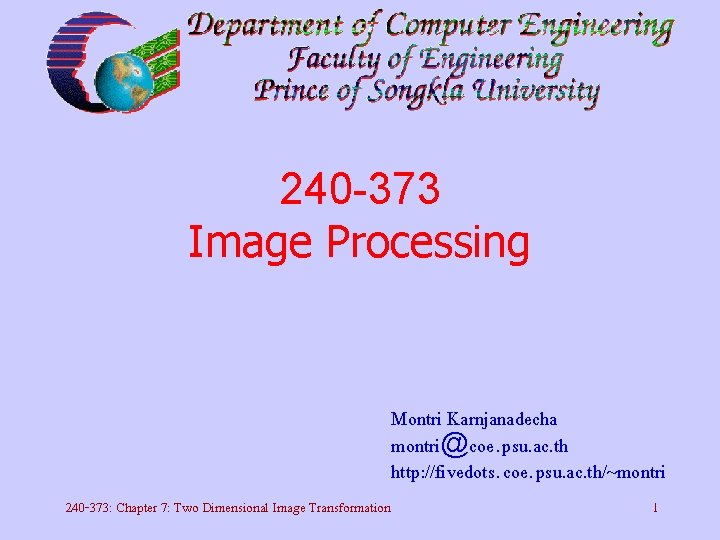240 -373 Image Processing Montri Karnjanadecha [email protected] psu. ac. th http: //fivedots. coe. psu. ac. th/~montri 240 -373: Chapter 7: Two Dimensional Image Transformation 1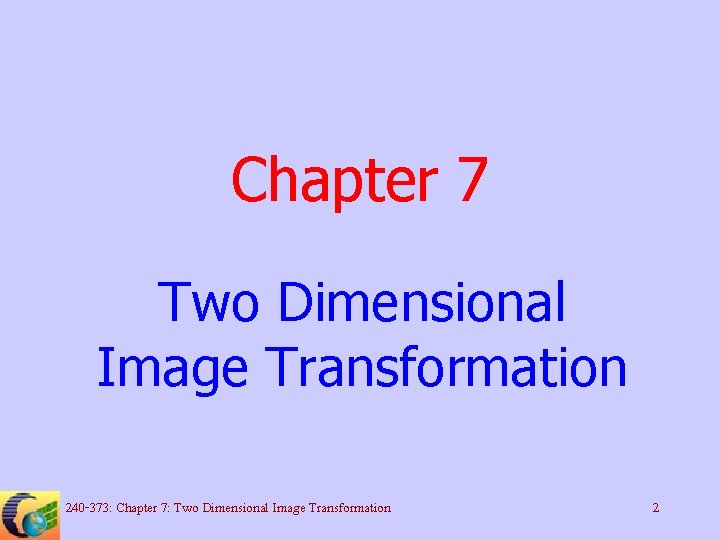Chapter 7 Two Dimensional Image Transformation 240 -373: Chapter 7: Two Dimensional Image Transformation 2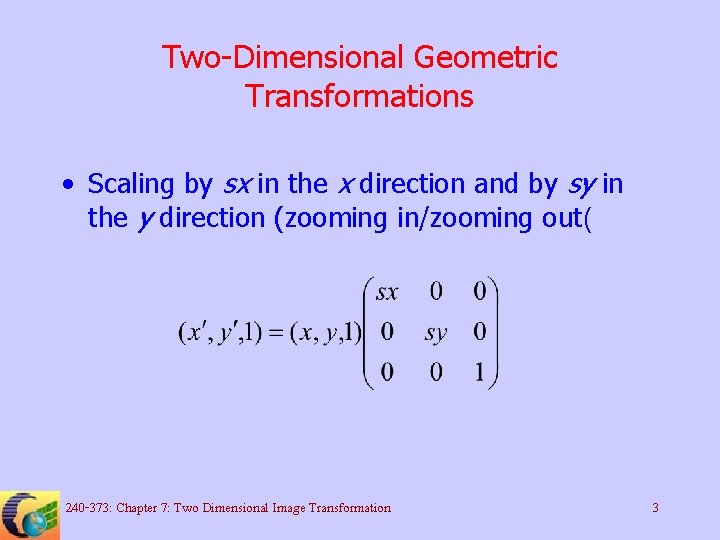Two-Dimensional Geometric Transformations • Scaling by sx in the x direction and by sy in the y direction (zooming in/zooming out( 240 -373: Chapter 7: Two Dimensional Image Transformation 3Two-Dimensional Geometric Transformations • Translating by tx in the x direction and by ty in the y direction 240 -373: Chapter 7: Two Dimensional Image Transformation 4Two-Dimensional Geometric Transformations • Rotating by a radian clockwise 240 -373: Chapter 7: Two Dimensional Image Transformation 5Inverse transformations • Scaling by sx in the x direction and by sy in the y direction (zooming in/zooming out( 240 -373: Chapter 7: Two Dimensional Image Transformation 6Inverse transformations • Translating by tx in the x direction and by ty in the y direction 240 -373: Chapter 7: Two Dimensional Image Transformation 7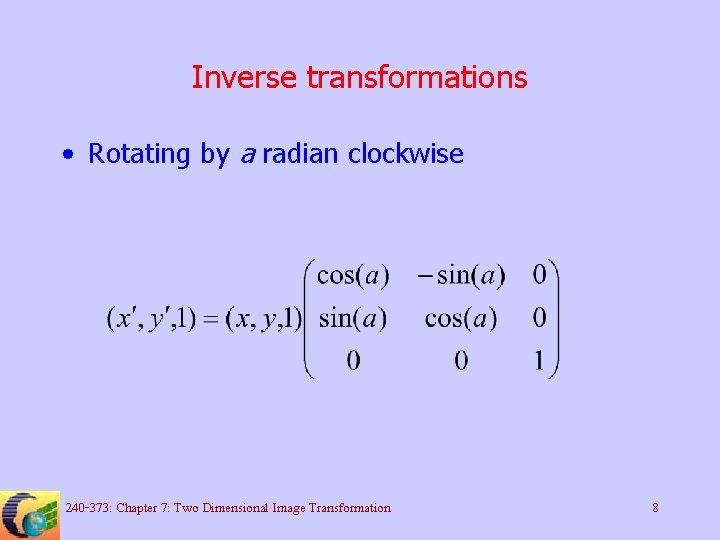Inverse transformations • Rotating by a radian clockwise 240 -373: Chapter 7: Two Dimensional Image Transformation 8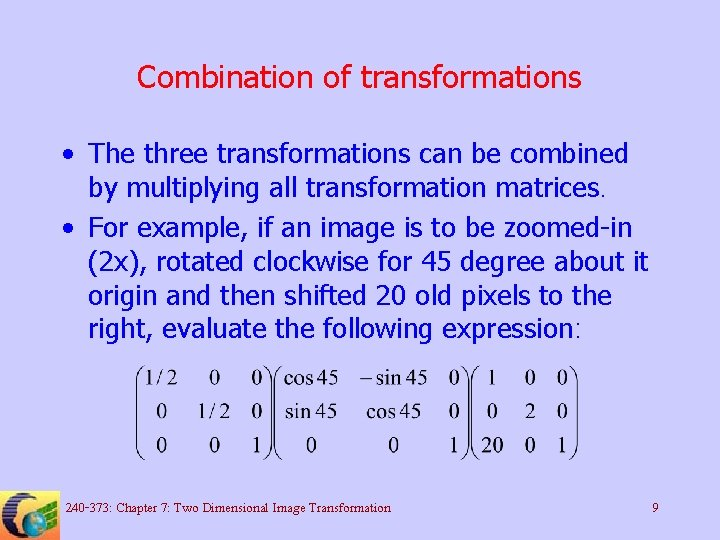Combination of transformations • The three transformations can be combined by multiplying all transformation matrices. • For example, if an image is to be zoomed-in (2 x), rotated clockwise for 45 degree about it origin and then shifted 20 old pixels to the right, evaluate the following expression: 240 -373: Chapter 7: Two Dimensional Image Transformation 9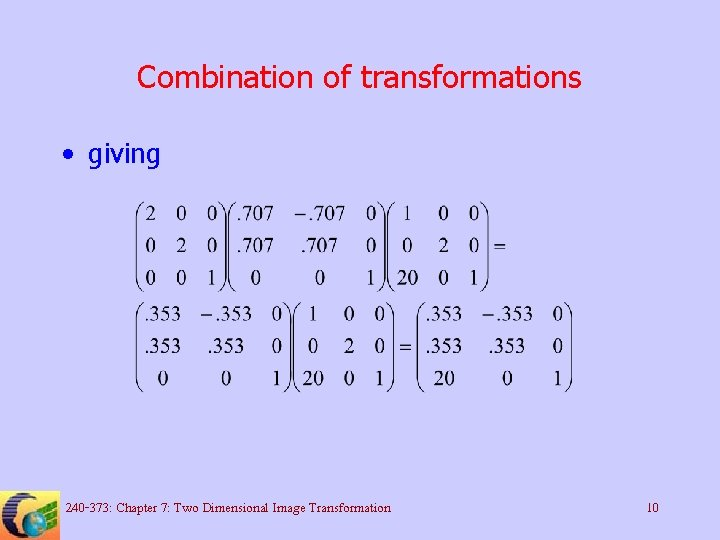Combination of transformations • giving 240 -373: Chapter 7: Two Dimensional Image Transformation 10Combination of transformations • For new pixel position (8, 10( new pixel will be at (26, 1( 240 -373: Chapter 7: Two Dimensional Image Transformation 11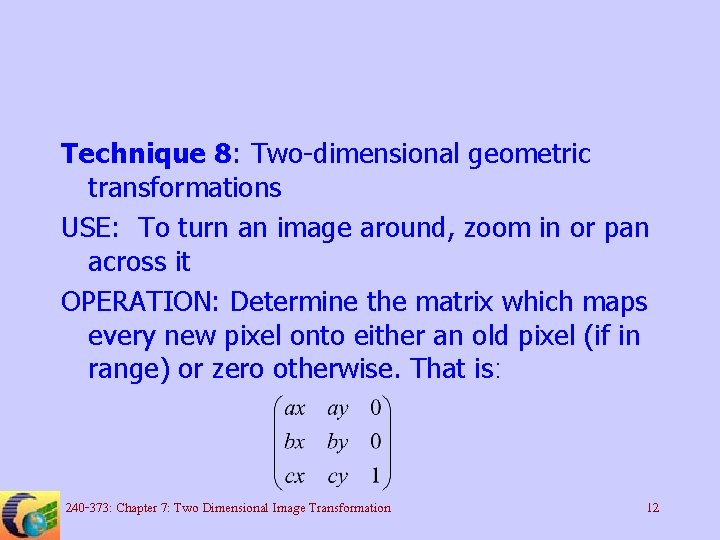Technique 8: Two-dimensional geometric transformations USE: To turn an image around, zoom in or pan across it OPERATION: Determine the matrix which maps every new pixel onto either an old pixel (if in range) or zero otherwise. That is: 240 -373: Chapter 7: Two Dimensional Image Transformation 12Starting a pixel (0, 0), plotting left to right, calculate atx = cx; aty = cy and make Increment x and atx = atx + ax; aty = aty + ay 240 -373: Chapter 7: Two Dimensional Image Transformation 13and repeat Inew allocation. Repeat for each x in the row. For the next row, set starting values to atx = bx*y + cx; aty = by*y + cy 240 -373: Chapter 7: Two Dimensional Image Transformation 14